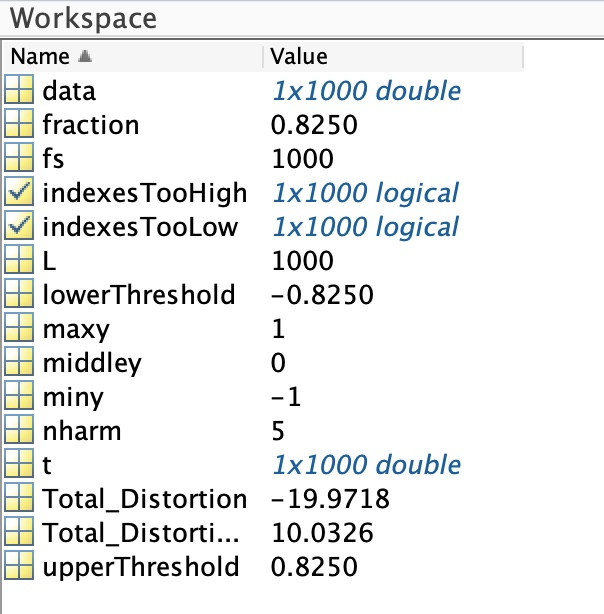Search

# Calculating Total Harmonic Distortion (THD) with Matlab

Updated: Feb 12

## Introduction

Calculating total harmonic distortion (THD) is one of the fundamental steps taken by an audio engineer when evaluating an audio product or system. Processing audio in analogue or digital domain introduces undesired distortion no matter how linear and accurate the system is. Quantifying such distortion is necessary in order to understand if the system is at the acceptable level.

Recently, while working on a DSP based audio system for a client, we've stumbled upon the need to measure THD in the digital audio workstation we were working on, which is based on Logic Pro X, Izotope RX and a few plugins. As it turns out, there is a plethora of built-in functions in these tools. There are also stacks of third-party plugins such as the ones from Waves Audio. But while they cover parameters such as LUFS and RMS, we could not find one that measures THD.

So we've decided to use Matlab to perform the calculations.

## Creating a baseline for measurement

The first step was to establish a process independently from the audio files capturing the output of the system we wanted to measure. Keep in mind that THD measurements depend on evaluating the amplitude of harmonics introduced by the non-linearity of the system you are measuring. So the best approach is to use a signal with a single harmonic as your input.

The code below creates a simple sine wave.

%% Data

% Create a signal sampled at 1kHz

t = 0:0.001:1-0.001;

data = 1*cos(2*pi*100*t);

L = length(data);

fs = 1000;

Now of course if you measure the THD of the signal above it will be virtually zero, only subject to the mathematical accuracy of Matlab itself... so let's introduce some clipping.

%% Clipping

% Clipping Data

maxy = max(data);

miny = min(data);

middley = (miny + maxy)/2;

% Determine top point as, say X% ofthe way from the middle line to the max

fraction = 0.825;

upperThreshold = middley + fraction * (maxy - middley);

lowerThreshold = middley - fraction * (middley - miny);

% Now clip

indexesTooHigh = data > upperThreshold;

data(indexesTooHigh) = upperThreshold;

indexesTooLow = data < lowerThreshold;

data(indexesTooLow) = lowerThreshold;

Plot the data.

%% Plots

% Plot Waveform

figure(1)

plot(1000*t(1:L),data(1:L))

title('Audio Data')

xlabel('t (milliseconds)')

ylabel('X(t)')Sine Wave Plot

Then calculate both the total distortion attenuation (in dB) and the total distortion factor (in %). The thd function is included in the signal processing toolbox in Matlab.

%% Calcs

% THD with 5 harmonics including fundamental

nharm = 5;

Total_Distortion = thd(data,fs,nharm);

Total_Distortion;

% THD factor in percent

Total_Distortion_Factor = 100*10^(Total_Distortion/20);

With the above you obtain total distortion of -19.97 dB or 10.03%Sine Wave Data

Try changing the clipping factor (fraction) to see how it affects the distortion.

## Enter the WAV file

This is where we switched to the WAV files from the system we are measuring, instead of the made up sine wave. This WAV file was captured at the highest specification our system allowed (32 bits, 48kHz, mono) and is the result of passing a -20 dBFS sine wave at 1kHz through it.

To load a WAV file in Matlab you have to use the 'Import data' function and then select the data and sampling frequency (fs) from the data it contains.

The code to handle the data is this.

%% Data

% Create Data importing wave file into 'data' and fs into 'fs'

T = 1/fs; % Sampling period

L = length(data); % Length of signal from data loaded (WAV)

t = (0:L-1)*T; % Time vector

Plotting the data is quite similar.

%% Plots

% Plot Waveform

figure(1)

plot(1000*t(1:L),data(1:L))

title('Audio Data')

xlabel('t (milliseconds)')

ylabel('X(t)')WAV File Plot

And the calculations are quite straightforward and similar to what we had done in the baseline file.

%% Calcs

% THD with 5 harmonics including fundamental

nharm = 5;

Total_Distortion = thd(data,fs,nharm);

Total_Distortion;

% THD factor in percent

Total_Distortion_Factor = 100*10^(Total_Distortion/20);WAV File Data

## Conclusion

In a world evolving more and more towards in-the-box audio work, it's important to know all the measurement tools you have at your disposal. Matlab is a great resource to bridge gaps not covered by plugins in your DAW.

Of course if you do find plugins which measure THD please drop us a line. Still, with Matlab you have full control of the measurement, and once you get the above process established, the measuring process is quite easy.